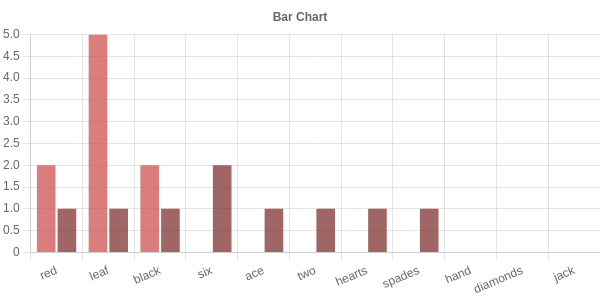# Count Constructors

rascal-0.33.7

#### Synopsis​

Generic function that can count constructors in a value of any algebraic data type.

#### Description​

In Colored Trees, we have seen a function that can count the number of red nodes in a `ColoredTree`. Is it possible to define a function that can count constructors in a value of any algerbaic data type?

We exploit the subtype relation (see Static Typing) between algebraic data typess and the type node to achieve this.

In real applications this becomes relevant when counting, for instance, statement types in programs.

#### Examples​

``import Node;import Map;data ColoredTree     = leaf(int N)          | red(ColoredTree left, ColoredTree right)     | black(ColoredTree left, ColoredTree right)    ;                 ColoredTree CT = red(black(leaf(1), red(leaf(2),leaf(3))), black(leaf(3), leaf(4)));data Suite     = hearts()     | diamonds()     | clubs()     | spades()    ;data Card     = two(Suite s)     | three(Suite s)     | four(Suite s)     | five(Suite s)     | six(Suite s)     | seven(Suite s)     | eight(Suite s)     | nine(Suite s)     | ten(Suite s)     | jack(Suite s)     | queen(Suite s)     | king(Suite s)     | ace(Suite s)    ;             data Hand = hand(list[Card] cards);Hand H = hand([two(hearts()), jack(diamonds()), six(hearts()), ace(spades())]);// Count frequencies of constructorsmap[str,int] count(node N) {      ❶    freq = ();        ❷    visit(N) {        ❸      case node M: {       name = getName(M);      ❹        freq[name] ? 0 += 1;     }  }  return freq;      ❺  }map[str,int] countRelevant(node N, set[str] relevant) = domainR(count(N), relevant);      ❻  test bool tstCount() =  count(CT) == ("red":2, "leaf":5, "black":2);test bool tstCountRelevant() = countRelevant(CT, {"leaf"}) == ("leaf" : 5);``

Two data types are introduced `ColoredTree` and `Hand` together with an example value of each (`CT`, respectively, `H`).

• ❶ The function `count` is defined.

• ❷ Introduces an empty map to maintain the frequencies.

• ❸ Defines a visit of argument `N`; it traverses the complete value of `N`.

• ❹ Defines the case that we encounter a node and we update its frequency count. First the name of the constructor is retrieved (using getName) and then the frequency is updated. The isDefined operator is used to provide a default value of 0 when the name was not yet in the map.

• ❺ The map `freq` is returned as result.

• ❻ Defines a variant `countRelevant`; it gets is an extra argument of relevant constructors names that is used to filter the map that is returned by `count` using domainR.

``rascal>count(CT);map[str, int]: ("red":2,"leaf":5,"black":2)rascal>count(H);map[str, int]: ("six":1,"ace":1,"two":1,"hearts":2,"spades":1,"hand":1,"diamonds":1,"jack":1)rascal>countRelevant(H, {"hearts", "spades"});map[str, int]: ("hearts":2,"spades":1)``

Comparing the two counts visually:

``rascal>import vis::Charts;okrascal>import Relation;okrascal>barChart(["CT", "H"], toRel(count(CT)), toRel(count(H)))``